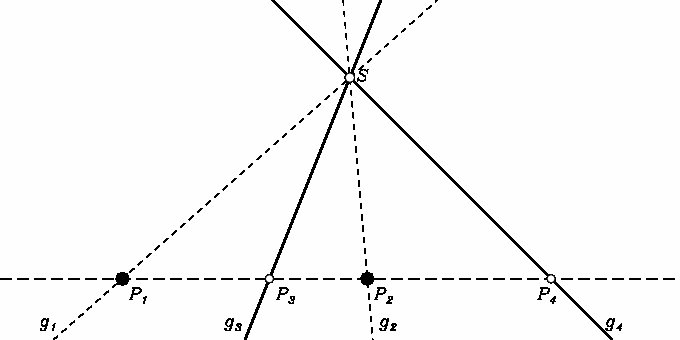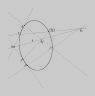## The cross ratio

is first of all a relation between a pair of points P1, P2 and a pair of lines g3, g4. In homogeneous coordinates, it has the value

D[P1,P2;g3, g4] =
(<g3P1>/<g3P2>)/(<g4P1>/<g4P2>)

and is projectively invariant. When one understands the lines as connection of points P3, P4 with some point S, we obtain a value for two pairs of points, which does not depend on the choice of S, when P3, P4 are collinear with P1, P2. When one understands the points P1, P2 as intersection of lines g1, g2 with some line s, we obtain a value for two pairs of lines, which does not depend on the choice of s, when g1, g2 are concurrent with g3, g4.

 Kapitel Portal